Today, I'm going to discuss a very important topic of Quantitative aptitude i.e. Sequence and Series. Sequence and Series is a mathematical concept and basically it is a logical concept.

## Sequence and Series

Sequence and Series problems just follow a particular pattern. Usually, series completion problems are asked in most of the quantitative aptitude exams. You just need to study the set relationship to complete the series. Once you recognize the pattern of the series, you will be able to solve the problem.

Remember, Study the set relationship first

In quantitative aptitude exam, four or five options are given, to make the questions easy, always try to eliminate the options. This will help you to save your precious time. Coming to the point, I'm going to discuss some standard patterns of series. Go through these rules and try to solve the problems related to this.

## Some standard patterns of series

Some number (or pattern of numbers) may be added or subtracted in each term to get the next term.

Example1: Study the following series and try to find the next term,

3, 6, 9,12,15, ?

Solution: This is very simple pattern. You can easily find that 3 is added to each term , and we are getting next term.

3+ 3= 6
6+ 3= 9
9+ 3= 12
Similarly, 15+ 3= 18. So, 18 will the next term.

Example2: Complete the following series

4, 6, 9, 13, 18,?

Solution: Pattern used in series:

4+ 2= 6
6+ 3= 9
9+ 4= 13
13+ 5= 18
Therefore, 18+ 6= 24. So, 24 will be next term.

Example3: Study one more term and find the next term of series.

13, 11, 9, 7, 5, ?

Solution: As this is decreasing pattern, you can easily find that 2 is subtracted from each term to get the next term.

13- 2= 11
11- 2= 9
9- 2= 7
Similarly, 5- 2= 3. So, 3 will be  the last term.

### Multiplication or Division

Another pattern may be related to multiplication or division of some number to each term to get the next term.

Example4: Study the pattern of series and try to find next term

4, 8, 24, 96,?

Solution: A particular pattern of numbers is multiplied to each term of series

4 times 2 = 8
8 times 3 = 24
24 times 4 = 96
So, 96 times  5 = 480. 480 will be the last term.

Example5: Complete the following series

480, 96, 24, 8,?

Solution: Study the pattern and note that it is a decreasing pattern and a particular series of number is divided from previous term

480/ 5 = 96
96/ 4 = 24
24/3 = 8
Continuing, 8/2= 4. Therefore, 4 will be the next term.

### Squaring or cubing

Another rules may be of squaring or cubing of the terms of series.

Example6: Complete the following series

4,9,16,25,?

Solution: This is a very simple pattern i.e. a square of some pattern of numbers

2^2= 4
3^2= 9
4^2= 16
5^2= 25
Continuing, 6^2= 36. 36 is the next term.

Example7: Study the following series and try to complete

1, 27, 125, 343,?

Solution: The series is based on following pattern:

1^3= 1
3^3= 27
5^3= 125
7^3= 343
Continuing, 9^3= 729, So, 729 will be the next term.

### Mixed Patterns

In some questions, combination of above discussed patterns are used. For example, some number is multiplied with first term of series and then some other number is subtracted to get the next term. I will give you some examples for more understanding. Firstly try to solve it yourself.

Example8: Try to find the next term of following series:

1, 2, 6, 21, 88,?

Solution: Study this series, you will find out that more than one rule is applied on it. Following pattern is used in it:

1 times 1 + 1 = 2
2 times 2 + 2 = 6
6 times 3 + 3 = 21
21 times 4 + 4 = 88
Series (1, 2, 3....) multiplied to term and then same series is added to get the next term
Therefore, 88 times 5 + 5 = 445. 445 is the next term.

Example9: Find the next term of following series:

276, 140, 68, 36,?

Solution: Following pattern is used in the series:

276/2 +2 = 140 (Term is divided by 2 then added by 2)
140/2 -2 = 68 (Term is divided by2 then subtracted by 2)
68/2 +2 = 36
Therefore, each term is divided by 2 then alternately added and subtracted by 2 to get the next term.
So 36/2 - 2 = 16. 16 is the next term.

Thus, there are large number of techniques to form number of series. The only thing is the pattern of the series.

Check some examples of Series: Sequence and Series examples
Soon, I will update some questions for practice.

Tricks to solve problems related to Series in Quantitative AptitudeReviewed by Jasleen Behl on Wednesday, January 15, 2014 Rating: 5

1.recently i found your such a good website admin! please post quantitative aptitude, reasoning shortcuts! thanks by ganesh

•Thnku, Will soon update.

2.in example 4, the term 96 is written as 69

•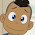yet it is still not fixed...!

•check clearly, fixed from so long time..

3.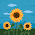EXCELLENT ARTICLE !!

•Thnku,, Soon I will update very informative articles, so be in touch

4.excellent website

5.Sir will you plz solve this question
1;2;8;33;148;..........;4626? An:765. I don't no logic behind it plz help mr

6.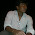1*1+1^2 = 2 2*2 + 2^2 = 8 8*3+3^2 = 33 33*4+ 4^2 = 148 148*5 + 5^2 = 765

7.3, 4,7, 11,...,.... I've to find next two terms, I guess, they are 18, 29 but doubt, options are a) 16,23 b) 18,29 c) 17,24 d) 19,27

•3+1=4; 4+3=7; 7+4=11; 11+7=18; 18+11=29

8.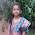1, 3, 8, 28, ?

Answer is 106. But I don't know how it has come. Please explain it.

9.2,9,28,65,126,217,344.Define the pattern.

•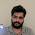2³+1=9
3³+1=28
4³+1=65 & so on..

10.Actually in the series there must be 26 instead of 28.
1*1+2=3
3*2+2=8
8*3+2=26
26*4+2=106

11.Actually in the series there must be 26 instead of 28.
1*1+2=3
3*2+2=8
8*3+2=26
26*4+2=106

I will try to respond asap